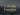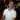# LARGE() Function in Excel

July 26, 2017

Sometimes you need to take a set of data and get the first, second, or third top values. You can do that with the LARGE() function in Excel. Here’s a quick article to help explain how to use the LARGE() function.

## LARGE() Function Defined

``LARGE(array, k)``

The `LARGE()` function will take an array and return the k-th largest value from the data. You can think of this as Excel sorting the data from largest to smallest and then you can say “give me the top largest item” by setting `k` to `1` and the formula would return the highest value from the list.

Parameter Definition
`array` The data to search the largest values from.
`k` This is the number that you give the formula to say “I was the top largest number” (which you would use `1`). If you wanted the second highest value, you would use `2` for `k`.## Large() Function Example

For this example we’ll be using the following spreadsheet:

You can also copy and paste this table into Excel to follow along:

Random Data More Random Data
92 87
16 79
46 69
63 77
4 47
38 90
74 35
58 89
3 87

### Finding the Top N Largest Value

To find the top largest value in the data, we can use this formula:

``=LARGE(\$A\$2:\$B\$10,1)``

This will return `92`.

Here is the formula breakdown:

``=LARGE(\$A\$2:\$B\$10, 1)``

If we expand out the array `\$A\$2:\$B\$10`, we get the following:

``=LARGE({92,87;16,79;46,69;63,77;4,47;38,90;74,35;58,89;3,87}, 1)``

Then Excel sorts the list from largest to smallest

``=LARGE({92,90,89,87,87,79,77,74,69,63,58,47,46,4,38,35,3,16}, 1)``

Finally, Excel takes the k-th largest value. In this case, it’s `1`, so it takes the `92` from the list and returns that.

If we want to find the second largest value, change the `k` parameter to `2`:

``=LARGE(\$A\$2:\$B\$10, 2)``

This returns `90`.

Wow, you read the whole article! You know, people who make it this far are true learners. And clearly, you value learning. Would you like to learn more about Excel? Please consider supporting me by buying me a coffee (it takes a lot of coffee to write these articles!).Written by Joseph who loves teaching about Excel.